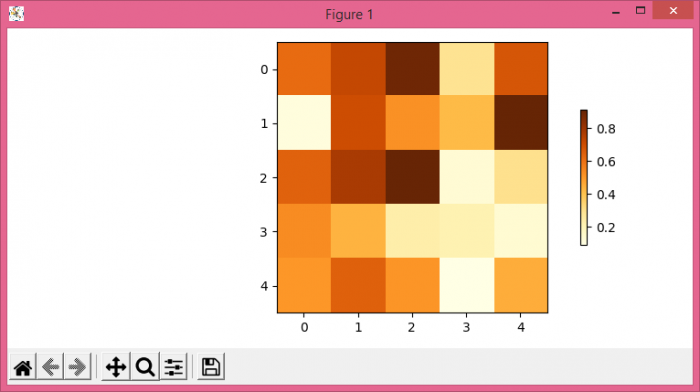# How to decrease colorbar width in Matplotlib?

MatplotlibPythonData Visualization

To decrease colorbar width in Matplotlib, we can use shrink in colorbar() method.

## Steps

• Set the figure size and adjust the padding between and around the subplots.
• Create a random data using numpy.
• Display the data as an image, i.e., on a 2D regular raster, with data.
• Create a colorbar for a ScalarMappable instance, im.
• To display the figure, use show() method.

## Example

import numpy as np
from matplotlib import pyplot as plt

plt.rcParams["figure.figsize"] = [7.50, 3.50]
plt.rcParams["figure.autolayout"] = True

data = np.random.rand(5, 5)
im = plt.imshow(data, cmap="YlOrBr")

plt.colorbar(im, shrink=0.50)

plt.show()

## Output